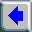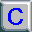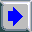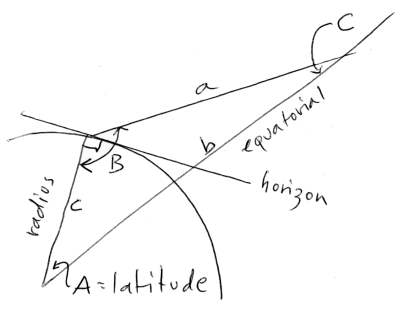## [Printed book in 3 volumes, plus Pdf, Epub, Mobi.]## Recovering the Lost World, A Saturnian Cosmology -- Jno Cook Appendix M: The Red Sirius.\$Revision: 42.7 \$ (sir.php)
Contents of this Appendix: [Ptolemy] [Sima Qian and Yu Ji-cai] [Gregory of Tours] [Al-Sufi] [The Maya] [Summary]

## Ptolemy, AD 200

In the second century AD, Ptolemy (working from Alexandria in northern Egypt) reports the star Sirius as red, as do others in late antiquity. However, Sirius is listed as white by the Arab (Persian) astronomer Al-Sufi in the Book of fixed Stars, published in AD 964. Both Ptolemy and Al-Sufi were very competent astronomers.

At a much earlier time Sirius was clearly recognized as white. As the brightest star in the sky, ancient records appropriately speak of Sirius as "the star of Venus," where Venus was the brightest planet in the sky. This would not make much sense if Sirius were inherently red.

Before 685 BC, as developed in the text, Sirius was located only a few degrees below the equatorial, rising almost directly east and setting almost directly west. It would have traveled above the Absu, which nowhere reached the height of the equatorial in the south skies. For example, at Cairo, at 30 degrees latitude (0.13 degrees south of Alexandria), Sirius would reach its highest point in the south sky at an elevation of about 61 degrees before 685 BC.

My estimate of the limit of the intact Absu was 8500 miles (14,000 km) above the surface of the Earth at the equator. This was seen at about 48 degrees above the south horizon at Cairo. At this time Sirius would have been white, since it did not shine through the Absu or the remaining red ring after the fall of the Absu.

In 685 BC the location of the equatorial shifted, so that at Cairo Sirius was lowered to 43 degrees at its highest point in the south sky. It now rose in the southeast, and set in the southwest. Sirius was now reported as "red," which means it appeared behind the last remnant ring of the Absu. From this information at Cairo we can locate the last red ring.[Image: Sight line to red ring. Illustration by J. Cook.]

The elevation of the red ring above the equator of the Earth can be found from the relationship:

a / sin(A) = b / sin(B) = c / sin(C).

From the diagram above, the location of the ring above the Earth's equator can be found from the radius of Earth and the two adjacent angles, where angle A is the latitude of Cairo, angle C is between the sight line and the extension of a radius line at the equator.

A = 30 degrees, the latitude of Giza
B = 90 + 43 = 133 degrees
C = 180 - B - A = 180 - (90 + 43) - 30 = 17 degrees.

In the following, b is the height of the ring from the center of the Earth and c is the radius of the Earth. Thus:

b = c * ( sin(C) / sin(B) ),
b = 4000 * ( sin(133) / sin(17) ) = 10,005 miles.

The last red ring is thus located 10,005 - 4000 = 6,000 miles (9,600 km) above the equator.

Ptolemy, I should note, actually could have had it both ways. For although Sirius would have been seen as red when it stood high in the sky, it rose in the southeast, away from the red snake in the sky (which touched the eastern horizon directly east), and set in the southwest, also away from the red ring. At these two locations it would have been white.

But in rising higher in the sky Sirius crossed the remaining red ring. In fact, at the latitude of Cairo I would presume that Sirius spent most of its travels behind the red ring.

## Sima Qian and Yu Ji-cai, AD 100 and 200

The change of color also shows up in Chinese documents, as, for example, in the Book of Asterisms by Sima Qian, dating from about 100 BC, which reads:

"If Wolf [Sirius] shows horns or changes colour, then there will be much banditry,"

or, from Secret Garden of the Observatory by Yu Ji-cai, of circa AD 200:

"If the Wolf Star shows rays or horns, or shakes about, or changes colour, then there will be war."

This information is from Jiang Xiao-yuan, in "The colour of Sirius as recorded in ancient Chinese texts" (Chinese Astronomy and Astrophysics, 1993), which sets out to prove that Sirius is noted as being white in four records, each dealing with color standards for stars, dated between 100 BC and AD 650, which were judged by Jiang to be more reliable than the astrological texts quoted above. Jiang admits, however,

"Although ancient Chinese writings always had a tradition of copying what previous authors had said, so that the four items listed [in the article] are probably correlated to some degree, we should not therefore deny ancient Chinese astrologers of any originality whatever. That [the "Jinzhou Book of Prognostication" of circa AD 100] lists both Sirius and Vega as standards for white star is worth noting. These two are indeed white bright stars of the same type."

However, the "shaking," "horns," and "changes in color" would be explained if Sirius, the brightest star in the sky, traveled behind a portion of the last remaining ring -- "shaking" being caused by the passage of the moving ring in front of the star (like with Saturn, the Earth's equatorial rings move at rotational speeds different from the planet). The "horns" are caused by refraction by the striation pattern of the intervening ring (the rings of the Absu had been described as reeds growing in a swamp and as bushes, thus radially oriented), and a "color change" is caused by entry and exit of Sirius from behind the ring.

This last is caused, as noted above, by the fact that Sirius is "attached" to the dome of the stars and thus travels in a circle with the polar axis as its center, whereas the rings are an artifact of the Earth, forming a crescent shape in the sky which is gathered together at the east and west cardinal location and spreads apart at its highest point in the south skies.

A look at where Sirius and the red ring were located in the sky when seen from Beijing might help. Beijing is at 39.5 degrees north latitude. Thus the equatorial rose 50.5 degrees up from the south horizon. With Sirius placed at a declination of 16.3 degrees (circa AD 150), it rose to a maximum height of 34.2 degrees.

As before, the length of the sight line is:

a = sqrt (10000^2 + 4000^2 - 2 * 10000 * 4000 * cos(39.5)).

This is 7367 miles. From this the angle of elevation to the rings can be found as:

sin(C) = (4000 / 7367) * sin(39.5) = 0.345.

From sine tables this is equal to an angle of 20.2 degrees. The elevation is thus 180 -39.5 -20.2 -90 = 30.3 degrees. This is 3.9 degrees below the highest location of Sirius in the south sky. Thus Sirius moved across the red ring twice each night.

## Gregory of Tours, AD 550

In about AD 560-590, Gregory of Tours still calls Sirius "reddish," although he may have copied that information from Ptolemy. Tours is at 47.38 degrees north latitude. At Tours the equatorial would be seen at an elevation of 90 degrees less the latitude, 90 - 47.38 = 42.6 degrees. In AD 550 Sirius would be seen at an angle of about 16 degrees below the equatorial (this is true everywhere for this date and for the early part of the first millennium AD), thus at 26.6 degrees.

Using the same geometry as before, we can find the distance between the observer and the intersection of the equatorial at the location of the remaining ring from:

a = sqrt (b^2 + c^2 - 2 * b * c * c(A))
a = sqrt (10000^2 + 4000^2 - 2 * 10000 * 4000 * cos(47.38).

This is 7863 miles. The angle C can be found from:

sin(C) = (c / a) * sin(A)
sin(C) = (4000 / 7863) * sin(47.38) = 0.3743.

The corresponding angle (from sine tables) is 22 degrees. Subtracting this angle and the latitude from 180 degrees yields 110.6 degrees. Now subtracting 90 degrees (the angle between the Earth radius and the horizontal), determines that the red ring would have been seen at an elevation of 20.6 degrees.

This is a considerable span -- 4.6 degrees -- below the culmination of Sirius. The path of Sirius would thus cross the red ring, and Sirius would turn red twice a night.

## Al-Sufi, AD 900

When, after about AD 900, the Arab astronomer Al-Sufi catalogues stars, he lists Sirius as "white," as it still is today. Al-Sufi worked from Isfahan at 33.63 degrees north latitude. At Isfahan the elevation of the equatorial is at 56.4 degrees, and Sirius travels (at about AD 900) at 15.4 degrees below the equatorial, and thus culminates at an elevation of 41 degrees above the south horizon.

Using the same geometry as above, the distance from the observer to the location of the ring (assuming it existed still) at the plane of the equatorial can be found from:

a = sqrt (10000^2 + 4000^2 - 2 * 10000 * 4000 * cos(33.6).

This is 7026 miles. From this the angle of elevation to the rings can be found as:

sin(C) = (4000 / 7026) * sin(33.6) = 0.315.

This represents an angle of 18.3 degrees. The elevation is thus 180 -33.6 -18.3 -90 = 38.1 degrees. This is only 2.9 degrees below the culmination of Sirius in the south sky. It suggests that, although at this location Sirius would have crossed the red ring if it still existed, by AD 900 the ring probably had disappeared. This is also suggested from the Maya construction project in AD 800. On the other hand, as an astronomer, perhaps Al-Sufi realized that the white star Sirius just passed through the red ring twice each day.

## Maya, AD 800

After about AD 800, when the Maya start the construction of a 61-mile (98 km) causeway running from east to west across the Northern Yucatan, this last red ring had most likely disappeared. It may, in fact, have been gone before AD 600. In the 20th century AD, the Maya still recalled the "cord in the sky," through which blood flowed, but which had disappeared in the past. The 16th-century AD Popol Vuh calls it the "river of blood." It can be assumed that the "river of blood" was like the "river of pus" (the ecliptic, as in the Popol Vuh) and that the "river" probably did not subtend more than a few degrees in the sky.

An investigation by George Jones in about AD 1910 at Quito, Peru, determined a width of the ecliptic as 2 to 3 degrees. The earliest iconography of the Olmecs at La Venta, dating to around 600 BC, also show the "Saint Andrew's Cross," the crossing of the ecliptic and equatorial in the night skies at the time of the equinoxes, as two bands of nearly the same width.

## Summary

Let me summarize the data, presented mostly in historical order, and adding Rome (AD 200) and Beijing (AD 150).

 ``` Intersection of Sirius with the red ring Sirius Sirius ring location era latitude equatorial declination elevation elevation diff -------- --- -------- ---------- ----------- --------- --------- ----- Cairo 200 30.0 deg 60.0 deg 16.3 deg 43.0 deg 43.0 deg 0.0 deg Beijing 150 39.5 50.5 16.3 34.2 30.3 3.9 Rome 200 41.9 48.1 16.5 31.6 27.3 4.3 Tours 570 47.4 42.6 16 26.6 22.0 4.6 Isfahan* 950 33.6 56.4 15.4 41.0 (38.1) 2.9 *-- Sirius listed as white at this time Declination of Sirius depends on the date ```

Since 685 BC, the path of Sirius through the sky always starts and ends about 19 degrees south of directly east and west. Thus the semi-circle described by the path of Sirius could fall completely within the semi-circle of the rings, but would cut through the band of the red ring and perhaps rise above it when directly in the south. Only at Cairo does the upper portion of the path of Sirius coincide with the ring -- but that is how I have defined this condition.

It is, of course, arbitrary, but justified in that many other authors from late antiquity note the redness of Sirius, including Babylonian cuneiform tablets after about 650 BC (at 33.3 degrees latitude) and Roman authors (at 41.9 degrees north latitude). The results for Rome in about AD 200 are much the same as for Beijing at the same date. I have added Rome to the list above.

Since Rome is at 41.9 degrees latitude, it could be suggested that the red band in the sky in circa AD 200 extended across 4 degrees in the sky. Four degrees is the equivalent of two hand-spans (without the thumbs) at arm's length. This measure might also be suggested by two other data points. First, the records of planetary actions in Babylon after 650 BC seem to indicate rather sudden changes in refractive patterns, suggesting a tight band of red. Second, the Quiche Popol Vuh call the red band a "river." The details of that story are contemporaneous with the plasma event of 685 BC.

I would expect Al-Sufi at Isfahan (33.6 latitude) to have seen a red-colored Sirius, for the latitude of Isfahan falls between Cairo and Rome, except that the date is obviously late. A hundred years earlier the Yucatan Maya were rebuilding the red road in the sky on Earth -- which almost guarantees that the red ring had disappeared within the previous hundred years or so.

In Beijing (39.5 degrees latitude), Sirius could have been the measure for white, for it is very bright, but could also legitimately described as changing color -- since it would have traveled behind the red ring in reaching its culmination. This is, in fact, true for all the sites listed above.

Calculations are in Unix bc notation, where ^ denotes exponentiation; the functions a(rctangent), s(ine), and c(osine) use radians; angle conversions to radians or degrees by the divisors rad=.017+ and deg=57.2+; other functions are shown as f( ); tan( )=s( )/c( )
units: million == 1,000,000; billion == 1,000,000,000;
AU == 93,000,000 miles.